# Using weights to calculate the difference in rating

You are here:

Sometimes Weights are used as part of a calculation. They give different scores to the responses, so you can convert a variable code into another value for calculation.

You can use weights to subtract the negative from the positive values of a rating scale variable. By using this data in a statistics table, you can see whether the ratings were generally positive or negative.

This example assumes a five-code scale, in which the middle value is neutral, such as Q6a (speed of service). Because the rating scale only contains counts of responses, you need to set up a derived variable in order to calculate the statistics of the scored response.

1. Click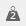to display the Weights window.
2. Click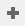to add a new weight and specify the Weight Details as follows:
• Name: WT6
• Label: Weight pos/neg
• Decimal places: 0
• Number of codes: 5
3. Specify the Code Details:
1. Clickto save the Weight.
2. Click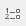to display the Variables window.
3. Clickto add a new variable.
4. Specify the Variable Details:
• Name: V10
• Label: Calculated difference
• Type: Derived (the variable will derive its data from other existing variables).
• Response: Quantity (the response will be a numeric value for each case).
5. Specify the Code Details to combine the Weight with the Speed of service rating variable:
1. Click on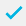to save the variable.
2. Click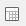to display the Analysis Definition dialog to create a table.
3. Specify the Analysis as V10, which is the variable to be weighted.
4. Select Statistics table from the list for the Break (or enter STATS).
5. Click OK and the table will be built.

The Sum will be the difference between the positive and negative values.

Contents